Point

サマリー

The point object is used frequently with cursors. Point features return a single point object instead of an array of point objects. All other feature types—polygon, polyline, and multipoint—return an array of point objects or an array containing multiple arrays of point objects if the feature has multiple parts.

説明

A Point is not a geometry class, but is commonly used to construct geometry. In the example below, a Point is used to create a PointGeometry object.

point = arcpy.Point(25282, 43770)
ptGeometry = arcpy.PointGeometry(point)

構文

Point ({X}, {Y}, {Z}, {M}, {ID})
 パラメーター 説明 データ タイプ X The X coordinate of the point. (デフォルト値は次のとおりです 0.0) Double Y The Y coordinate of the point. (デフォルト値は次のとおりです 0.0) Double Z The Z coordinate of the point. (デフォルト値は次のとおりです None) Double M The M value of the point. (デフォルト値は次のとおりです None) Double ID The shape ID of the point. (デフォルト値は次のとおりです 0) Integer

プロパティ

 プロパティ 説明 データ タイプ ID(読み書き) An integer used to uniquely identify the point. Integer M(読み書き) The measure value of the point. Double X(読み書き) The horizontal coordinate of the point. Double Y(読み書き) The vertical coordinate of the point. Double Z(読み書き) The elevation value of the point. Double

方法の概要

 方法 説明 clone (point_object) Clone the Point object. contains (second_geometry, {relation}) Indicates if the base geometry contains the comparison geometry. contains is the opposite of within. Only True relationships are shown in this illustration.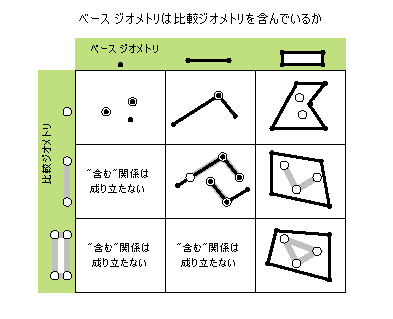crosses (second_geometry) Indicates if the two geometries intersect in a geometry of a lesser shape type. Two polylines cross if they share only points in common, at least one of which is not an endpoint. A polyline and an polygon cross if they share a polyline or a point (for vertical line) in common on the interior of the polygon which is not equivalent to the entire polyline. Only True relationships are shown in this illustration.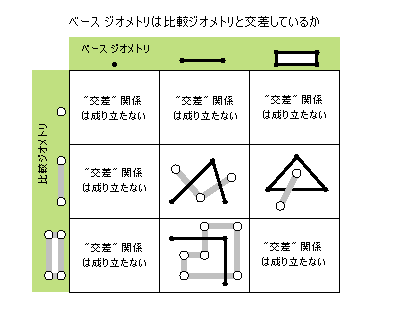disjoint (second_geometry) Indicates if the base and comparison geometries share no points in common. Two geometries intersect if disjoint returns False. Only True relationships are shown in this illustration.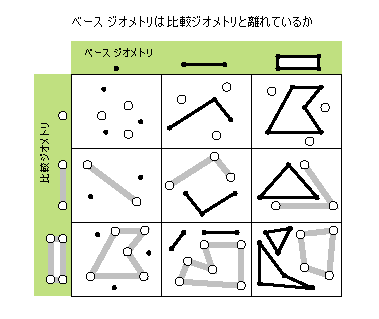equals (second_geometry) Indicates if the base and comparison geometries are of the same shape type and define the same set of points in the plane. This is a 2D comparison only; M and Z values are ignored.Only True relationships are shown in this illustration.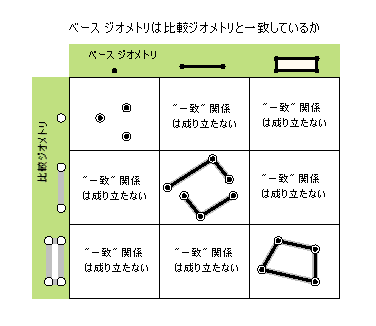overlaps (second_geometry) Indicates if the intersection of the two geometries has the same shape type as one of the input geometries and is not equivalent to either of the input geometries. Only True relationships are shown in this illustration.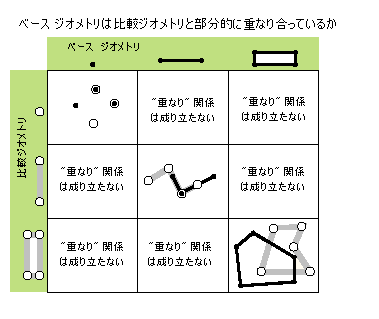touches (second_geometry) Indicates if the boundaries of the geometries intersect. Two geometries touch when the intersection of the geometries is not empty, but the intersection of their interiors is empty. For example, a point touches a polyline only if the point is coincident with one of the polyline end points. Only True relationships are shown in this illustration.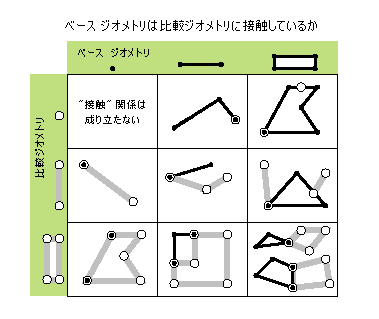within (second_geometry, {relation}) Indicates if the base geometry is within the comparison geometry. within is the opposite operator of contains. Only True relationships are shown in this illustration.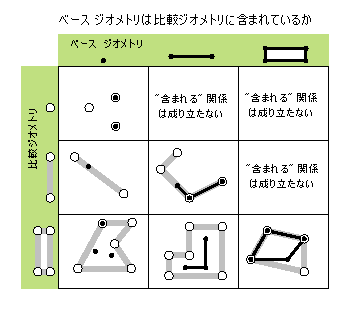The base geometry is within the comparison geometry if the base geometry is the intersection of the geometries and the intersection of their interiors is not empty. within is a Clementini operator, except in the case of an empty base geometry.

方法

clone (point_object)
 パラメーター 説明 データ タイプ point_object A Point object. Point
contains (second_geometry, {relation})
 パラメーター 説明 データ タイプ second_geometry A second geometry. Object relation The spatial relationship type.BOUNDARY — Relationship has no restrictions for interiors or boundaries.CLEMENTINI — Interiors of geometries must intersect. Specifying CLEMENTINI is equivalent to specifying None. This is the default.PROPER — Boundaries of geometries must not intersect.(デフォルト値は次のとおりです None) String

 データ タイプ 説明 Boolean A return Boolean value of True indicates this geometry contains the second geometry.
crosses (second_geometry)
 パラメーター 説明 データ タイプ second_geometry A second geometry. Object

 データ タイプ 説明 Boolean A return Boolean value of True indicates the two geometries intersect in a geometry of a lesser shape type.
disjoint (second_geometry)
 パラメーター 説明 データ タイプ second_geometry A second geometry. Object

 データ タイプ 説明 Boolean A return Boolean value of True indicates that the two geometries share no points in common.
equals (second_geometry)
 パラメーター 説明 データ タイプ second_geometry A second geometry. Object

 データ タイプ 説明 Boolean A return Boolean value of True indicates that the two geometries are of the same shape type and define the same set of points in the plane.
overlaps (second_geometry)
 パラメーター 説明 データ タイプ second_geometry A second geometry. Object

 データ タイプ 説明 Boolean A return Boolean value of True indicates the intersection of the two geometries has the same dimension as one of the input geometries.
touches (second_geometry)
 パラメーター 説明 データ タイプ second_geometry A second geometry. Object

 データ タイプ 説明 Boolean A return Boolean value of True indicates the boundaries of the geometries intersect.
within (second_geometry, {relation})
 パラメーター 説明 データ タイプ second_geometry A second geometry. Object relation The spatial relationship type.BOUNDARY — Relationship has no restrictions for interiors or boundaries.CLEMENTINI — Interiors of geometries must intersect. Specifying CLEMENTINI is equivalent to specifying None. This is the default.PROPER — Boundaries of geometries must not intersect.(デフォルト値は次のとおりです None) String

 データ タイプ 説明 Boolean A return Boolean value of True indicates this geometry is contained within the second geometry.

コードのサンプル

Point example

Create Point object and display its properties.

import arcpy

# Create point object
point = arcpy.Point(2000, 2500)

# Print point properties
print("Point properties:")
print(" ID: {0}".format(point.ID))
print(" X:  {0}".format(point.X))
print(" Y:  {0}".format(point.Y))
Point example 2

Examine point objects in polygon array object, returned from geometry object.

import arcpy

# Create cursor to retrieve Hawaii shape
feature_class = "c:/data/Hawaii.shp"
cursor = arcpy.da.SearchCursor(feature_class, ["SHAPE@"])

for row in cursor:
# Get the geometry object from the shape field
print("Number of Hawaiian islands: {0}".format(row.partCount))

# GetPart returns an array of point objects for each part.
for island in row.getPart():
print("Vertices in island: {0}".format(island.count))
for point in island:
print("X: {0}, Y: {1})".format(point.X, point.Y))